GeeksforGeeks App
Open AppBrowser
Continue

## Related Articles

• CBSE Class 11 Chemistry Notes

A redox reaction is a sort of chemical reaction in which the oxidation states of atoms are changed and electrons are exchanged between the two involved reactants. It is a reaction involving electron transport that combines two reactions: oxidation and reduction. The oxidation states of the reactive chemical species are changed in this reaction, with one chemical species losing electrons and the other chemical species gaining electrons at the same time.

Every aspect of life needs a sense of equilibrium. It’s also not a good idea to eat too much chocolate. Even reactions must be balanced. The balancing of redox reactions is an essential component of chemistry. What are redox reactions, and how do they work? Iron rusts as a result of a redox process. Other redox reactions may be found all around us. Let’s learn more about them and examine the steps involved in redox reaction balance.

### Redox Reaction

The term “redox reaction” refers to a reaction in which both oxidation and reduction occur at the same time.

What is meant by Oxidation?

• The Addition of oxygen to a substance is called Oxidation.
• The Removal of hydrogen from a substance is also called Oxidation.

For Example- 2Cu + O2 → 2Cuo

In this reaction, oxygen is added to copper. Therefore, copper is OXIDISED.

What is meant by reduction Reaction?

• The Addition of hydrogen to a substance is called reduction.
• The Removal of oxygen from a substance is also called reduction.

For Example- CuO  +  H2  → Cu  +  H2O

In this reaction, Oxygen is removed from copper oxide. Therefore, copper oxide (CuO) is REDUCED.

The gain of electrons is known as reduction, and the loss of electrons is known as oxidation. Redox reaction/redox process refers to the combination of reduction and oxidation reactions. As stated previously, understanding “balancing redox reactions” is essential.

In a redox process, there are commonly two techniques for balancing redox reactions (chemical equations). The Oxidation Number Method and the Half-Reaction Method are the two types of methods.

### Balancing of Redox Reactions

Chemical equations for redox processes are balanced using two ways. One technique is based on the oxidation number of the reducing and oxidizing agents changing, while the other method is based on splitting the redox reaction into two half-reactions, one involving oxidation and the other involving reduction. Both of these ways are in use, and it is up to the individual who uses them to decide which one to utilize.

Two methods are used to balance the redox reaction. One of these methods is based on the Oxidation Number Method and the second one is based on Half Reaction Method.

Oxidation Number Method

The composition and formulas for the reactants and products must be known when creating equations for oxidation-reduction processes, just as they must for other reactions. The oxidation number method is best demonstrated in the steps that follow:

There are two mediums, Acidic Medium and Basic Medium.

Steps to Balance the Redox Reaction in Acidic Medium by Oxidation Number Method :

Step 1: Assign the oxidation number to all elements in the reaction to identify atoms that change oxidation number during the reaction.

How to find an Oxidation Number?

1. The oxidation number of an atom or molecule is 0 (Zero). Eg, Cu, N2.
2. Sum of oxidation number in compound is 0. eg, CuO : Oxidation number (O.N.) of Cu = +2 and O.N. of O is -2. ∴ +2 + (-2) = 0.
3. More Electronegative atom = -ve O.S.
4. Less Electronegative atom = +ve O.S.

Examples: To find oxidation number.

• Cr2O72-

2(x)+7(-2)=-2, (Here, x is O.N. of Cu and -2 is O.N. of O)

∴2x-14=-2

∴2x=-2+14

∴x=6 (Oxidation number of Cu)

• Na3PO3

3(+1)+(x)+3(-2)=0

∴3+x-6=0

∴x=+3 (Oxidation number of P)

Step 2: Identify the elements/atoms which undergo oxidation and reduction.

Step 3: After balancing atoms that have undergone oxidation and reduction, balance charge by cross multiplication.

Step 4: Balance all other atoms except oxygen and hydrogen.

Step 5: Add H2O to balance the oxygen.

Step 6: By adding H+ molecules to the reactants or products, you can make the number of hydrogen atoms in the expression on both sides equal.

Let us have been using the example below to explain the steps involved in the method.

Example: Balance the Redox Reaction in Acidic Medium by Oxidation Number Method.

P  +  HNO3   →   HPO3  +  NO  +  H2O

Solution:

Step 1: Assign the oxidation number to all elements in the reaction to identify atoms that change oxidation number during the reaction.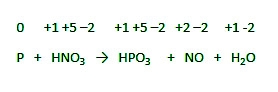Step 2: Identify the elements/atoms which undergo oxidation and reduction.

Oxidation: P  →  HPO3

Reduction: HNO3   →   NO

Step 3: After balancing atoms that have undergone oxidation and reduction, balance charge by cross multiplication.

P  +  HNO3   →   HPO3  +  NO  +  H2O

There is an increase in the O.N. of P from 0 to +5 which is an increase of 5 in O.N. and there is a decrease in the O.N. of N from +5 to +2 which is a decrease of 3 in O.N.

Cross multiplication: 3P  +  5HNO3   →   3HPO3  +  5NO  +  H2O

Step 4: Balance all other atoms except oxygen and hydrogen.

3P  +  5HNO3   →   3HPO3  +  5NO  +  H2

Step 5: Add H2O to balance the oxygen.

3P  +  5HNO3   →   3HPO3  +  5NO  +  H2

Step 6: By adding H+ molecules to the reactants or products, you can make the number of hydrogen atoms in the expression on both sides equal.

3P  +  5HNO3   →   3HPO3  +  5NO  +  H2O

Hence, the equation is balanced.

Steps to Balance the Redox Reaction in Basic Medium by Oxidation Number Method :

Step 1: Assign the oxidation number to all elements in the reaction to identify atoms that change oxidation number during the reaction.

Step 2: Identify the elements/atoms which undergo oxidation and reduction.

Step 3: Balance all other atoms except oxygen and hydrogen.

Step 4: After balancing atoms that have undergone oxidation and reduction, balance charge by cross multiplication.

Step 5: Add H2O to balance the oxygen.

Step 6: By adding H+ molecules to the reactants or products, you can make the number of hydrogen atoms in the expression on both sides equal.

Step 7: Add as many OH ions on both sides as a number of H+ ions on one side.

Let us have been using the example below to explain the steps involved in the method.

Example: Balance the Redox Reaction in Basic Medium by Oxidation Number Method.

Cl2  +  IO3  +  OH   →   IO4  +  Cl  +  H2O

Solution:

Step 1: Assign the oxidation number to all elements in the reaction to identify atoms that change oxidation number during the reaction.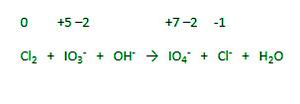Step 2: Identify the elements/atoms which undergo oxidation and reduction.

Oxidation: IO3  →  IO4

Reduction: Cl2  →  Cl

Step 3: Balance all other atoms except oxygen and hydrogen.

Cl2  +  IO3  +OH   →   IO4  +  2Cl  +  H2O

Step 4: After balancing atom that have undergone oxidation and reduction, balance charge by cross multiplication.

Cl2  +  IO3  +OH   →   IO4  +  2Cl  +  H2O

There is an increase in the O.N. of I from +5 to +7 that is an increase of 2 in O.N. and there is a decrease in the O.N. of Cl from 0 to -2 that is a decrease of 2 in O.N.

Cancel both difference (2) to each other.

Cl2  +  IO3  +OH   →   IO4  +  2Cl  +  H2O

Step 5: Add H2O to balance the oxygen.

Cl2  +  IO3   + H2O   →   IO4  +  2Cl

Step 6: By adding H+ molecules to the reactants or products, you can make the number of hydrogen atoms in the expression on both sides equal.

Cl2  +  IO3  + H2O   →   IO4  +  2Cl  +  2H+

Step 7: Add as many OH- ions on both sides as number of H+ ions on one side.

Cl2  +  IO3  + H2O  +  2OH   →   IO4  +  2Cl  +  2H+  +  2OH

∴ Cl2  +  IO3  + H2O  +  2OH   →   IO4  +  2Cl  +  2H2O

∴ Cl2  +  IO3  +  2OH   →   IO4  +  2Cl  +  ( 2H2O – H2O )

∴  Cl2  +  IO3  +  2OH   →   IO4  +  2Cl  +  H2O

Hence, the equation is balanced.

Half Reaction Method (Ion Electron Method) :

The two half equations are balanced individually and then combined to create a balanced equation in this method.

There are two mediums: Acidic Medium and Basic Medium

Steps to Balance the Redox Reaction in Acidic Medium by Half Reaction Method :

Step 1: In ionic form, generate an imbalanced equation for the reaction.

Step 2: Identify the elements/atoms which undergo oxidation and reduction.

Step 3: Break the reaction into two halves.

• Oxidation Half
• Reduction Half

Step 4: Balance the Half reaction.

• Balance all other atoms except oxygen and hydrogen.
• Add H2O to balance the oxygen.
• Balance Hydrogen (Add H+ ion) with protons.
• Balance the charge with the electron.

Step 5: To balance the charges, add electrons to one side of the half-reaction. If necessary, multiply one or both half-reactions by an appropriate value to make the number of electrons in the two half-reactions equal.

Step 6: To get the overall reaction, we combine the two half-reactions and cancel the common term on both sides. The net ionic equation is thus obtained.

Step 7: Check that both sides of the equation have the same kind and number of atoms, as well as the same charges. This final check confirms that the equation is perfectly balanced in terms of atoms and charges.

Example: Balance the Redox Reaction in Acidic Medium by Half Reaction Method.

Cr2O72-(aq)  +  HNO2(aq)  →  Cr3+(aq)  +  NO3(aq)

Solution:

Step 1: In ionic form, generate an imbalanced equation for the reaction.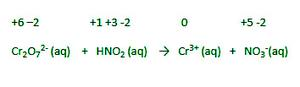Step 2: Identify the elements/atoms which undergo oxidation and reduction.

Oxidation: HNO2(aq)  →  NO3(aq)

Reduction: Cr2O72-(aq)  →  Cr3+(aq)

Step 3: Break the reaction in two halves.

Reduction Half,

Cr2O72-(aq)  →  Cr3+(aq)

Oxidation Half,

HNO2(aq)  →  NO3(aq)

Step 4: Balance the Half reaction.

• Balance all other atoms except oxygen and hydrogen.

(In 1st reaction, there are 2 moles of Cr on left side so we have to take 2 mole of Cr on right side.)

Cr2O72-(aq)  →  2Cr3+(aq)

(In 2nd reaction),

HNO2(aq)  →  NO3(aq)

• Add H2O to balance the oxygen.

Cr2O72-(aq)  →  2Cr3+(aq)  +  7H2O(l)

HNO2(aq)  +  H2O(l)  →  NO3(aq)

• Balance Hydrogen (Add H+ ion) with protons.

Cr2O72-(aq)  +  14H+(aq)  →   2Cr3+(aq)  +  7H2O(l)

HNO2(aq)  +  H2O(l)  →  NO3(aq)  +  3H+(aq)

• Balance the charge with electron.

In 1st reaction,

Cr2O72-(aq)  +  14H+(aq)  →   2Cr3+(aq)  +  7H2O(l)

LHS : (2-)  +  (14+) = (12+)charges

RHS : 2(3+) = (6+)charges

Therefore,

Cr2O72-(aq)  +  14H+(aq)  +  6e  →   2Cr3+(aq)  +  7H2O(l)

In 2nd reaction,

HNO2(aq)  +  H2O(l)  →  NO3(aq)  +  3H+(aq)

LHS : No charges

RHS : (1-) + (3+) = (2+)charges

Therefore,

HNO2(aq)  +  H2O(l)  →  NO3(aq)  +  3H+(aq)  +  2e

Step 5: To balance the charges, add electrons to one side of the half reaction. If necessary, multiply one or both half reactions by an appropriate value to make the number of electrons in the two half reactions equal.

Here, 6 electrons in 1st reaction and 2 electrons in 2nd reaction. so we multiply

1st reaction,

Cr2O72-(aq)  +  14H+(aq)  +  6e  →   2Cr3+(aq)  +  7H2O(l)

2nd reaction,

HNO2(aq)  +  H2O(l)  →  NO3(aq)  +  3H+(aq)  +  2e

∴ 3( HNO2(aq)  +  H2O(l)  →  NO3(aq)  +  3H+(aq)  +  2e )

∴ 3HNO2(aq)  +  3H2O(l)  →  3NO3(aq)  +  9H+(aq)  +  6e

Step 6: To get the overall reaction, we combine the two half reactions and cancel the common term on both sides. The net ionic equation is thus obtained.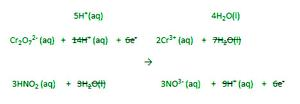Cr2O72-(aq)  +  5H+(aq)  +  3HNO2(aq)    →     2Cr3+(aq)  +  4H2O(l)  +  3NO3-(aq)

Step 7] Check that both sides of the equation have the same kind and number of atoms, as well as the same charges. This final check confirms that the equation is perfectly balanced in terms of atoms and charges.

Hence, the equation is balanced.

Steps to Balance the Redox Reaction in Basic Medium by Half Reaction Method :

Step 1: In ionic form, generate an imbalanced equation for the reaction.

Step 2: Identify the elements/atoms which undergo oxidation and reduction.

Step 3: Break the reaction into two halves.

• Oxidation Half
• Reduction Half

Step 4: Balance the Half reaction.

• Balance all other atoms except oxygen and hydrogen.
• Add H2O to balance the oxygen.
• Balance Hydrogen (Add H+ ion) with protons.
• Balance the charge with the electron.

Step 5: To balance the charges, add electrons to one side of the half-reaction. If necessary, multiply one or both half-reactions by an appropriate value to make the number of electrons in the two half-reactions equal.

Step 6: To get the overall reaction, we combine the two half-reactions and cancel the common term on both sides. The net ionic equation is thus obtained.

Step 7: Add as many OH- ions on both sides as a number of H+ ions on one side.

Step 8: Check that both sides of the equation have the same kind and number of atoms, as well as the same charges. This final check confirms that the equation is perfectly balanced in terms of atoms and charges.

Example: Balance the Redox Reaction in Basic Medium by Half Reaction Method.

MnO4  +  I   →   MnO2  +  I2

Solution:

Step 1: In ionic form, generate an imbalanced equation for the reaction.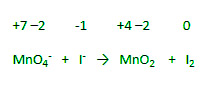Step 2: Identify the elements/atoms which undergo oxidation and reduction.

Oxidation: I   →   I2

Reduction: MnO4   →   MnO2

Step 3: Break the reaction in two halves.

Oxidation Half

I   →   I2

Reduction Half

MnO4–   →   MnO2

Step 4: Balance the Half reaction.

• Balance all other atoms except oxygen and hydrogen.

2I   →   I2

(Here, 2 moles of I on right side so we have to take 2 mole of I on left side.)

MnO4   →   MnO2

• Add H2O to balance the oxygen.

2I   →   I2

MnO4   →   MnO +  2H2O

• Balance Hydrogen (Add H+ ion) with protons.

2I   →   I2

MnO4  +  4H+  →   MnO2  +  2H2O

• Balance the charge with electron.

2I   →   I2  +  2e

MnO4  +  4H+  +  3e  →   MnO2  +  2H2O

Step 5: To balance the charges, add electrons to one side of the half reaction. If necessary, multiply one or both half reactions by an appropriate value to make the number of electrons in the two half reactions equal.

Here, 2 electrons in 1st reaction and 3 electrons in 2nd reaction. so we balance the electron.

3( 2I   →   I2  +  2e )

∴  6I   →   3I2  +  6e

2( MnO4  +  4H+  +  3e  →   MnO2  +  2H2O )

∴ 2MnO4  +  8H+  +  6e  →   2MnO2  +  4H2O

Step 6: To get the overall reaction, we combine the two half reactions and cancel the common term on both sides. The net ionic equation is thus obtained.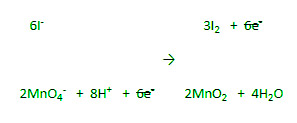Step 7: Add as many OH- ions on both sides as number of H+ ions on one side.

6I  +  2MnO4  +  8H+  +  8OH  →     3I2  +  2MnO2  +  4H2O  +  8OH

∴  6I  +  2MnO4  +  8H2O    →     3I2  +  2MnO2  +  4H2O  +  8OH

∴  6I  +  2MnO4  +  4H2O    →     3I2  +  2MnO2  +   8OH

Step 8: Check that both sides of the equation have the same kind and number of atoms, as well as the same charges. This final check confirms that the equation is perfectly balanced in terms of atoms and charges.

Hence, the equation is balanced.

### Sample Problems

Problem 1: Determine the Balance Redox Reaction in acidic medium by Oxidation number method for:

CuO  +  NH3   →  Cu  +  N2  +  H2O

Solution:

Step 1] Assign the oxidation number to all elements in the reaction to identify atoms which change oxidation number during the reaction.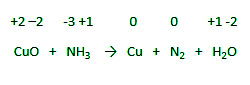Step 2] Identify the elements/atoms which undergo oxidation and reduction.

Oxidation:

NH3   →   N2

Reduction :

CuO   →  Cu

Step 3] After balancing atom that have undergone oxidation and reduction, balance charge by cross multiplication.

CuO  +  NH3   →  Cu  +  N2  +  H2O

There is an increase in the O.N. of N from -3 to 0 that is an increase of 3 in O.N. and there is a decrease in the O.N. of Cu from +2 to 0 that is a decrease of 2 in O.N.

Cross multiplication,

3CuO  +  2NH3   →  3Cu  +  2N2  +  H2O

Step 4] Balance all other atoms except oxygen and hydrogen.

3CuO  +  4NH3   →  3Cu  +  2N2  +  H2O

Step 5] Add H2O to balance the oxygen.

3CuO  +  4NH3   →  3Cu  +  2N2  +  H2O  +2H2O

Step 6] By adding H+ molecules to the reactants or products, you can make the number of hydrogen atoms in the expression on both sides equal.

3CuO  +  4NH3   →  3Cu  +  2N2  +  H2O  +2H2O  +  6H+

∴ 3CuO  +  2NH3   →  3Cu  +  N2  +  3H2

Hence, the equation is balanced.

Problem 2: Find the Balanced Redox Reaction in acidic medium by Ion electron method for:

Fe2+  +  Cr2O72-   →   Cr3+  +  Fe3+

Solution:

Step 1] In ionic form, generate an imbalanced equation for the reaction.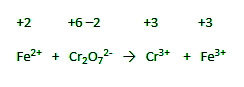Step 2] Identify the elements/atoms which undergo oxidation and reduction.

Oxidation,

Fe2+   →   Fe3+

Reduction,

Cr2O72-  →   Cr3+

Step 3] Break the reaction in two halves.

Oxidation Half,

Fe2+   →   Fe3+

Reduction Half,

Cr2O72-   →   Cr3+

Step 4] Balance the Half reaction.

• Balance all other atoms except oxygen and hydrogen.

Fe2+   →   Fe3+

Cr2O72-   →   2Cr3+

• Add H2O to balance the oxygen.

Fe2+   →   Fe3+

Cr2O72-   →   2Cr3+  +  7H2O

• Balance Hydrogen (Add H+ ion) with protons.

Fe2+   →   Fe3+

Cr2O72-  +  14H →   2Cr3+  +  7H2O

• Balance the charge with electron.

In 1st reaction,

Fe2+   →   Fe3+

LHS : (2+) charges

RHS : (3+)charges

Therefore,

Fe2+   →   Fe3+  +  e

In 2nd reaction,

Cr2O72-  +  14H+  →   2Cr3+  +  7H2O

LHS : (2-) + (14+) = (12+)charges

RHS : (6+) = (6+)charges

Therefore,

Cr2O72-  +  14H+  +  6e–   →   2Cr3+  +  7H2O

Step 5] To balance the charges, add electrons to one side of the half reaction. If necessary, multiply one or both half reactions by an appropriate value to make the number of electrons in the two half reactions equal.

(Here, 1 electrons in 1st reaction and 6 electrons in 2nd reaction. so we balance the electrons.)

1st reaction,

6( Fe2+   →   Fe3+  +  e )

∴ 6Fe2+   →   6Fe3+  + 6e

2nd reaction,

Cr2O72-  +  14H+  +  6e   →   2Cr3+  +  7H2O

Step 6] To get the overall reaction, we combine the two half reactions and cancel the common term on both sides. The net ionic equation is thus obtained.

6Fe2+  +  Cr2O72-  +  14H+  →   6Fe3+  +  2Cr3+  +  7H2O

Step 7] Check that both sides of the equation have the same kind and number of atoms, as well as the same charges. This final check confirms that the equation is perfectly balanced in terms of atoms and charges.

Hence, the equation is balanced.

Problem 3: Determine the Balanced Redox Reaction in the Basic medium by Half reaction method for:

Ag(s) + Zn2+(aq)   →   Ag2O(aq)  +  Zn(s)

Solution:

Step 1] In ionic form, generate an imbalanced equation for the reaction.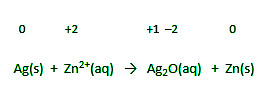Step 2] Identify the elements/atoms which undergo oxidation and reduction.

Oxidation,

Ag(s)  →  Ag2O(aq)

Reduction,

Zn2+(aq)  →  Zn(s)

Step 3] Break the reaction in two halves.

Oxidation Half,

Ag(s)  →  Ag2O(aq)

Reduction Half,

Zn2+(aq)  →  Zn(s)

Step 4] Balance the Half reaction.

• Balance all other atoms except oxygen and hydrogen.

2Ag(s)  →  Ag2O(aq)

Zn2+(aq)   →   Zn(s)

• Add H2O to balance the oxygen.

H2O(l)  +  2Ag(s)   →   Ag2O(aq)

Zn2+(aq)   →   Zn(s)

• Balance Hydrogen (Add H+ ion) with protons.

H2O(l)  +  2Ag(s)   →   Ag2O(aq)  +  2H+(aq)

Zn2+(aq)   →   Zn(s)

• Balance the charge with electron.

H2O(l)  +  2Ag(s)   →   Ag2O(aq)  +  2H+(aq)  +  2e

Zn2+(aq)  +  2e–   →   Zn(s)

Step 5] To balance the charges, add electrons to one side of the half reaction. If necessary, multiply one or both half reactions by an appropriate value to make the number of electrons in the two half reactions equal.

H2O(l)  +  2Ag(s)   →   Ag2O(aq)  +  2H+(aq)  +  2e

Zn2+(aq)  +  2e   →   Zn(s)

Step 6] To get the overall reaction, we combine the two half reactions and cancel the common term on both sides. The net ionic equation is thus obtained.

H2O(l)  +  2Ag(s)  + Zn2+ →   Ag2O(aq)  +  2H+(aq)  +  Zn(s)

Step 7] Add as many OH- ions on both sides as number of H+ ions on one side.

2Ag(s)  +  Zn2+(aq)  +  2OH(aq)   →   Zn(s)  +  Ag2O(aq)  +  H2O(l)

Step 8] Check that both sides of the equation have the same kind and number of atoms, as well as the same charges. This final check confirms that the equation is perfectly balanced in terms of atoms and charges.

Hence, the equation is balanced.

Problem 4: What is the Balanced Redox Reaction in acidic medium by Oxidation number method for:

Cu  +  NO3   →   NO2  +  Cu2+

Solution:

Step 1] Assign the oxidation number to all elements in the reaction to identify atoms which change oxidation number during the reaction.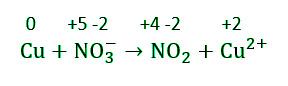Step 2] Identify the elements/atoms which undergo oxidation and reduction.

Oxidation,

Cu   →  Cu2+

Reduction,

NO3–    →   NO2

Step 3] After balancing atom that have undergone oxidation and reduction, balance charge by cross multiplication.

Cu  +  NO3   →   NO2  +  Cu2+

There is an increase in the O.N. of Cu from 0 to +2 that is an increase of 2 in O.N. and there is a decrease in the O.N. of N from +5 to +4 that is a decrease of 1 in O.N.

Cross multiplication,

Cu  +  2NO3   →   2NO2  +  Cu2+

Step 4] Balance all other atoms except oxygen and hydrogen.

Cu  +  2NO3   →   2NO2  +  Cu2+

Step 5] Add H2O to balance the oxygen.

Cu  +  2NO3   →   2NO2  +  Cu2+  +  2H2O

Step 6]  By adding H+ molecules to the reactants or products, you can make the number of hydrogen atoms in the expression on both sides equal.

Cu  +  2NO3  +  4H+   →   2NO2  +  Cu2+  +  2H2O

Hence, the equation is balanced.

Problem 5: Find the Balanced Redox Reaction in the Basic medium by Oxidation number method for:

[Cr(OH)4]  +  H2O2   →   CrO42-  +  H2O

Solution:

Step 1] Assign the oxidation number to all elements in the reaction to identify atoms which change oxidation number during the reaction.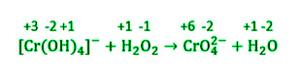Step 2] Identify the elements/atoms which undergo oxidation and reduction.

Oxidation,

[Cr(OH)4]    →  CrO42-

Reduction,

H2O2      →   H2O

Step 3] Balance all other atoms except oxygen and hydrogen.

[Cr(OH)4]  +  H2O2   →   CrO42-  +  H2O

Step 4] After balancing atom that have undergone oxidation and reduction, balance charge by cross multiplication.

There is an increase in the O.N. of chromium from +3 to +6 that is an increase of 3 in O.N. and there is a decrease in the O.N. of one oxygen atom in hydrogen peroxide from −1 to −2 that is a decrease of 1 in O.N.

Cross multiplication,

2[Cr(OH)4]–  +  3H2OCr2   →   CrO42-+  H2O

Step 5] Add H2O to balance the oxygen.

2[Cr(OH)4]–  +  3H2O2      2CrO42-  +  6H2O

Step 6] Add as many OH- ions on both sides as number of H+ ions on one side.

2[Cr(OH)4]  +  3H2O2  +  2OH   →  2CrO42-  +  8H2O

Hence the equation is balanced.

My Personal Notes arrow_drop_up
Related Tutorials